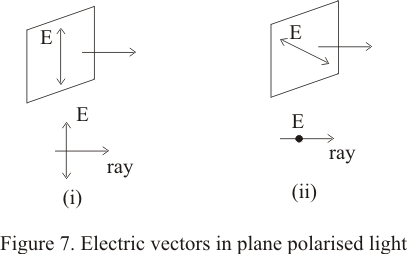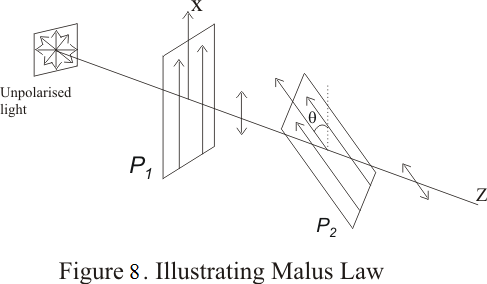# Diffraction and polarization of light

## 8) Polarization and electric vector

• we know that a transverse EM wave contains both electric and magnetic field and electric field in light wave is perpendicular to the direction of propagation of light wave

• We generally define direction of light vibration to be that of the electric vector E

• For an ordinary unpolarised beam electric vector keeps changing its direction in random manner

• when light is allowed to pass through a Polaroid the emergent light is plane polarized with its electric vector vibrating in a particular direction

• The direction of vector of emergent beam depends on the orientation of the Polaroid and the plane of polarization is designed as the plane containing E-vector and light ray

• Figure below shows the plane polarized light due to i) a vertical E-vector ii) a horizontal E-vector## 9)Law of Malus

• consider the figure given below in which an unpolarized light passes through a Polaroid P1 and then through Polaroid P2 making an angle θ with y ax-s• After light propagating along x-direction passes through the Polaroid P1 ,the electric vector associated with the polarized light will vibrate only along y-axis

• Now if we allow this polarized beam to again pass through a polarized P2 making an angle θ with y-axis then if E0 is the amplitude of incident electric field on P2 then amplitude of wave emerging from P2 would be E0cosθ and hence intensity of emerging beam would be

I=I0cos2θ                                 ---(4)

where I0 is the intensity of beam emerging from P2 when θ=0

• This equation (4) represents the law of malus

• Thus when a plane polarized light is incident on an analyzer ,the emerging light varies in accordance with the equation (4) where θ is the angle between the planes of transmission of the analyzer and the polarizer

## 10) Brewster's law

• Brewster law is a simple relationship between angle of maximum polarization and the refractive index of the medium

• Brewster�s law is given by relationship
μ=tani                                     --(5)

where i is the Polarizing angle
μis the index of refraction
It is clear from equation (5) that when light is incident at polarizing angle then reflected ray is at right angles to the reflected ray